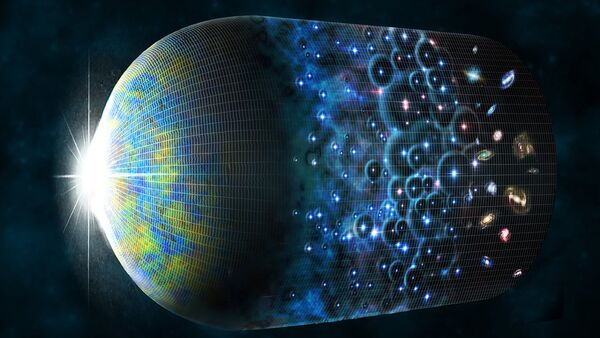Science

# Physics explained the Hubble constant and the age of the UniverseAmerican and British physicists, using the known distance from the Earth to fifty galaxies, said the value of the Hubble constant used in calculations of the age of the Universe, which, in accordance with the new data, is 12.6 billion years. The study is published in the journal Astronomical Journal.

To date, the time of the Big Bang that spawned the Universe, scientists estimate, using computer simulation, which is based on the distance to the oldest stars, the behavior of galaxies and the expansion rate of the Universe. The idea is to calculate how much time it will take for all the objects returned to the initial state.

A key parameter to calculate “the beginning” — the Hubble constant, a factor used by the American scientist Edwin Hubble first calculated the expansion rate of the Universe in 1929. This ratio relates the distance of extragalactic object — a galaxy or quasar with the speed of his removal.

A more modern method that uses to estimate the age of the Universe CMB — cosmic microwave background radiation uniformly fills the Universe, and emerged in the Big Bang, gives a different value of the Hubble constant and, consequently, a different age reference points.

Researchers from the US and the UK, led by the astronomer James Somerton (James Schombert) from the University of Oregon used an entirely new method of calculating distances in space, independent of the Hubble constant, and use based on empirical observations of the dependence of the Tully-Fisher linking the mass or luminosity of a spiral galaxy at a speed of rotation.

“The problem of scale distances, as we know, is incredibly difficult because distances to galaxies are huge, and the indicators of these ranges, weak and difficult to calibrate” — presented in a press release from the University of Oregon, the words of Sombart.

Using precisely defined distances to 50 galaxies as benchmarks, the authors calculated the distance of 95 other galaxies, and specified the corridor of the values of the relations Tully-Fisher. A more accurate view of the masses and speeds of the galaxies allowed us to mathematically obtain the age and expansion rate of the Universe.

According to the researchers, the Hubble constant — the expansion rate of the Universe is 75.1 ±2.3 kilometers per second per megaparsec, and the age of the Universe — about 12.6 billion years.

Traditional methods of calculations determine the values of the Hubble in district 75, and the background radiation is about 67. The authors write that “the value below 70 can be excluded with a 95 percent degree of certainty”.

Various methods, based on different values of the Hubble constant, estimate the age of the Universe between 12 billion and 14.5 billion years. For example, according to the spacecraft NASA WMAP (Wilkinson Microwave Anisotropy Probe), studying the CMB, this is the age of 13.77 billion years, and this figure is used in the standard model of cosmology the Big Bang.

“This difference is far beyond observational errors and causes a lot of friction in the cosmological community, indicating that our understanding of the physics of the Universe is incomplete,” — said Sombart.

A new study, partly based on observations with the space telescope “Spitzer”.

Source:

ria.ru

Check Also
Close